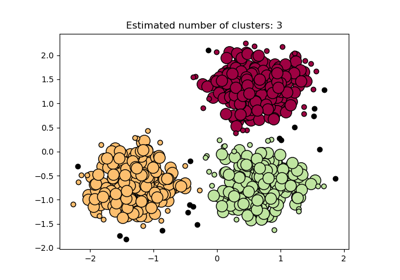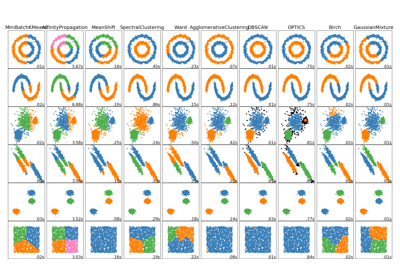# sklearn.cluster.DBSCAN¶

class sklearn.cluster.DBSCAN(eps=0.5, min_samples=5, metric=’euclidean’, metric_params=None, algorithm=’auto’, leaf_size=30, p=None, n_jobs=None)[source]

Perform DBSCAN clustering from vector array or distance matrix.

DBSCAN - Density-Based Spatial Clustering of Applications with Noise. Finds core samples of high density and expands clusters from them. Good for data which contains clusters of similar density.

Read more in the User Guide.

Parameters: eps : float, optional The maximum distance between two samples for one to be considered as in the neighborhood of the other. This is not a maximum bound on the distances of points within a cluster. This is the most important DBSCAN parameter to choose appropriately for your data set and distance function. min_samples : int, optional The number of samples (or total weight) in a neighborhood for a point to be considered as a core point. This includes the point itself. metric : string, or callable The metric to use when calculating distance between instances in a feature array. If metric is a string or callable, it must be one of the options allowed by sklearn.metrics.pairwise_distances for its metric parameter. If metric is “precomputed”, X is assumed to be a distance matrix and must be square. X may be a sparse matrix, in which case only “nonzero” elements may be considered neighbors for DBSCAN. New in version 0.17: metric precomputed to accept precomputed sparse matrix. metric_params : dict, optional Additional keyword arguments for the metric function. New in version 0.19. algorithm : {‘auto’, ‘ball_tree’, ‘kd_tree’, ‘brute’}, optional The algorithm to be used by the NearestNeighbors module to compute pointwise distances and find nearest neighbors. See NearestNeighbors module documentation for details. leaf_size : int, optional (default = 30) Leaf size passed to BallTree or cKDTree. This can affect the speed of the construction and query, as well as the memory required to store the tree. The optimal value depends on the nature of the problem. p : float, optional The power of the Minkowski metric to be used to calculate distance between points. n_jobs : int or None, optional (default=None) The number of parallel jobs to run. None means 1 unless in a joblib.parallel_backend context. -1 means using all processors. See Glossary for more details. core_sample_indices_ : array, shape = [n_core_samples] Indices of core samples. components_ : array, shape = [n_core_samples, n_features] Copy of each core sample found by training. labels_ : array, shape = [n_samples] Cluster labels for each point in the dataset given to fit(). Noisy samples are given the label -1.

OPTICS
A similar clustering at multiple values of eps. Our implementation is optimized for memory usage.

Notes

For an example, see examples/cluster/plot_dbscan.py.

This implementation bulk-computes all neighborhood queries, which increases the memory complexity to O(n.d) where d is the average number of neighbors, while original DBSCAN had memory complexity O(n). It may attract a higher memory complexity when querying these nearest neighborhoods, depending on the algorithm.

One way to avoid the query complexity is to pre-compute sparse neighborhoods in chunks using NearestNeighbors.radius_neighbors_graph with mode='distance', then using metric='precomputed' here.

Another way to reduce memory and computation time is to remove (near-)duplicate points and use sample_weight instead.

cluster.OPTICS provides a similar clustering with lower memory usage.

References

Ester, M., H. P. Kriegel, J. Sander, and X. Xu, “A Density-Based Algorithm for Discovering Clusters in Large Spatial Databases with Noise”. In: Proceedings of the 2nd International Conference on Knowledge Discovery and Data Mining, Portland, OR, AAAI Press, pp. 226-231. 1996

Schubert, E., Sander, J., Ester, M., Kriegel, H. P., & Xu, X. (2017). DBSCAN revisited, revisited: why and how you should (still) use DBSCAN. ACM Transactions on Database Systems (TODS), 42(3), 19.

Examples

>>> from sklearn.cluster import DBSCAN
>>> import numpy as np
>>> X = np.array([[1, 2], [2, 2], [2, 3],
...               [8, 7], [8, 8], [25, 80]])
>>> clustering = DBSCAN(eps=3, min_samples=2).fit(X)
>>> clustering.labels_
array([ 0,  0,  0,  1,  1, -1])
>>> clustering
DBSCAN(algorithm='auto', eps=3, leaf_size=30, metric='euclidean',
metric_params=None, min_samples=2, n_jobs=None, p=None)


Methods

 fit(self, X[, y, sample_weight]) Perform DBSCAN clustering from features or distance matrix. fit_predict(self, X[, y, sample_weight]) Performs clustering on X and returns cluster labels. get_params(self[, deep]) Get parameters for this estimator. set_params(self, \*\*params) Set the parameters of this estimator.
__init__(self, eps=0.5, min_samples=5, metric=’euclidean’, metric_params=None, algorithm=’auto’, leaf_size=30, p=None, n_jobs=None)[source]
fit(self, X, y=None, sample_weight=None)[source]

Perform DBSCAN clustering from features or distance matrix.

Parameters: X : array or sparse (CSR) matrix of shape (n_samples, n_features), or array of shape (n_samples, n_samples) A feature array, or array of distances between samples if metric='precomputed'. sample_weight : array, shape (n_samples,), optional Weight of each sample, such that a sample with a weight of at least min_samples is by itself a core sample; a sample with negative weight may inhibit its eps-neighbor from being core. Note that weights are absolute, and default to 1. y : Ignored
fit_predict(self, X, y=None, sample_weight=None)[source]

Performs clustering on X and returns cluster labels.

Parameters: X : array or sparse (CSR) matrix of shape (n_samples, n_features), or array of shape (n_samples, n_samples) A feature array, or array of distances between samples if metric='precomputed'. sample_weight : array, shape (n_samples,), optional Weight of each sample, such that a sample with a weight of at least min_samples is by itself a core sample; a sample with negative weight may inhibit its eps-neighbor from being core. Note that weights are absolute, and default to 1. y : Ignored y : ndarray, shape (n_samples,) cluster labels
get_params(self, deep=True)[source]

Get parameters for this estimator.

Parameters: deep : boolean, optional If True, will return the parameters for this estimator and contained subobjects that are estimators. params : mapping of string to any Parameter names mapped to their values.
set_params(self, **params)[source]

Set the parameters of this estimator.

The method works on simple estimators as well as on nested objects (such as pipelines). The latter have parameters of the form <component>__<parameter> so that it’s possible to update each component of a nested object.

Returns: self

## Examples using sklearn.cluster.DBSCAN¶Demo of DBSCAN clustering algorithmComparing different clustering algorithms on toy datasets